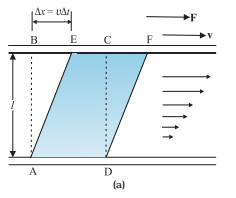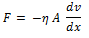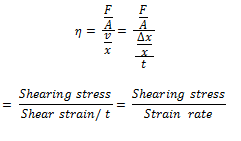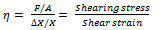Viscosity is the property of fluid by virtue of which an internal force of friction comes into play when a fluid is in motion and which opposes the relative motion between its different layers. The backward dragging force, called viscous drag or viscous force, acts tangentially on the layers of the fluid in motion and tends to destroy its motion.

Suppose we consider a fluid like oil enclosed between two glass plates as shown in Fig. 1(a). The bottom plate is fixed while the top plate is moved with a constant velocity v relative to the fixed plate. If oil is replaced by honey, a greater force is required to move the plate with the same velocity. Hence we say that honey is more viscous than oil. The fluid in contact with a surface has the same velocity as that of the surfaces. Hence, the layer of the liquid in contact with top surface moves with a velocity v and the layer of the liquid in contact with the fixed surface is stationary. The velocities of layers increase uniformly from bottom (zero velocity) to the top layer (velocity v). For any layer of liquid, its upper layer pulls it forward while lower layer pulls it backward.This results in force between the layers. This type of flow is known as laminar.Fig. 1(a) A layer of liquid sandwiched between two parallel glass plates in which the lower plate is fixed and the upper one is moving to the right with velocity v

## Short but important points about Viscosity:

• Viscosity is like friction and converts kinetic energy into heat energy.
• No fluid has zero viscosity.
• Thin liquids like water, alcohol etc. ; are less viscous than thick liquids coal tar, blood, honey, glycerin etc.
• Unlike solids, the strain in a flowing liquid increases with time continuously. So for solids with the elastic modulus of rigidity, the shearing stress is proportional to shear strain, while for fluids it is proportional to the time rate of change of strain or strain rate. The coefficient of viscosity of fluids can be defined as the ratio of shearing stress to the strain rate. Moreover,
• Newton’s formula for viscous force between two parallel layers is• Coefficient of viscosity,Modulus of rigidity,Thus the coefficient of viscosity of liquids is analogous to the modulus of rigidity of solids.

The SI unit of viscosity is poiseiulle (PL). Its other units are Nsm-2 or Pa s. The dimensions of viscosity are [ML-1T-1]. The coefficient of viscosity η is in poise.